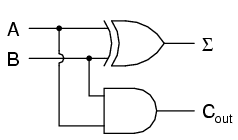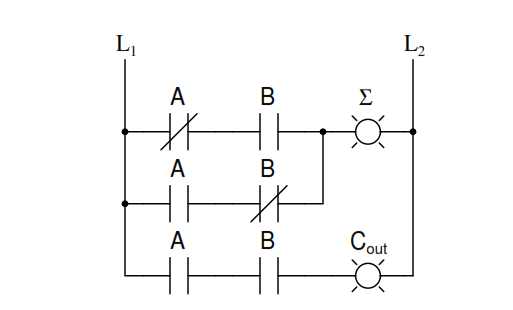You are here:

Suppose we wanted to build a device that could add two binary bits together. Such a device (A Binary Adder) is known as a half-adder, and its gate circuit looks like this:The Σ symbol represents the “sum” output of the half-adder, the sum\’s least significant bit (LSB). Cout represents the “carry” output of the half-adder, the sum\’s most significant bit (MSB).

If we were to implement this same function in ladder (relay) logic, it would look like this:Either circuit is capable of adding two binary digits together. The mathematical “rules” of how to add bits together are intrinsic to the hard-wired logic of the circuits.

If we wanted to perform a different arithmetic operation with binary bits, such as multiplication, we would have to construct another circuit. The above circuit designs will only perform one function: add two binary bits together. To make them do something else would take re-wiring, and perhaps different componentry.

In this sense, digital arithmetic circuits aren\’t much different from analog arithmetic (operational amplifier) circuits: they do exactly what they\’re wired to do, no more and no less.

We are not, however, restricted to designing digital computer circuits in this manner. It is possible to embed the mathematical “rules” for any arithmetic operation in the form of digital data rather than in hard-wired connections between gates. The result is unparalleled flexibility in operation, giving rise to a whole new kind of digital device: the programmable computer.

While this chapter is by no means exhaustive, it provides what I believe is a unique and interesting look at the nature of programmable computer devices, starting with two devices often overlooked in introductory textbooks: look-up table memories and finite-state machines.